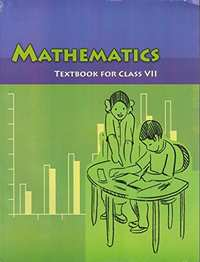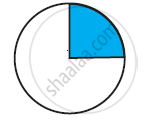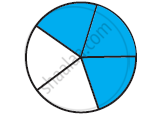CBSE Class 7CBSE
Share

NCERT solutions for Class 7 Mathematics chapter 8 - Comparing Quantities

Mathematics for Class 7

NCERT Mathematics Class 7Chapter 8: Comparing Quantities

Ex. 8.10Ex. 8.20Ex. 8.30

Chapter 8: Comparing Quantities Exercise 8.10 solutions [Page 157]

Ex. 8.10 | Q 1.1 | Page 157

Find the ratio of Rs 5 to 50 paise

Ex. 8.10 | Q 1.2 | Page 157

Find the ratio of 15 kg to 210 g

Ex. 8.10 | Q 1.3 | Page 157

Find the ratio of 9 m to 27 cm

Ex. 8.10 | Q 1.4 | Page 157

Find the ratio of 30 days to 36 hours

Ex. 8.10 | Q 2 | Page 157

In a computer lab, there are 3 computers for every 6 students. How many computers will be needed for 24 students?Ex. 8.10 | Q 3 | Page 157

Population of Rajasthan = 570 lakhs and population of UP = 1660 lakhs.

Area of Rajasthan = 3 lakh km2 and area of UP = 2 lakh km2.

1) How many people are there per km2 in both these States?

2) Which State is less populated?

Chapter 8: Comparing Quantities Exercise 8.20 solutions [Pages 164 - 165]

Ex. 8.20 | Q 1.1 | Page 164

Convert the given fractional numbers to per cents.

1/8

Ex. 8.20 | Q 1.2 | Page 164

Convert the given fractional numbers to per cents.

5/4

Ex. 8.20 | Q 1.3 | Page 164

Convert the given fractional numbers to per cents.

3/40

Ex. 8.20 | Q 1.4 | Page 164

Convert the given fractional numbers to per cents.

2/7

Ex. 8.20 | Q 2.1 | Page 165

Convert the given decimal fractions to per cents.

0.65

Ex. 8.20 | Q 2.2 | Page 165

Convert the given decimal fractions to per cents.

2.1

Ex. 8.20 | Q 2.3 | Page 165

Convert the given decimal fractions to per cents.

0.02

Ex. 8.20 | Q 2.4 | Page 165

Convert the given decimal fractions to per cents.

12.35

Ex. 8.20 | Q 3.1 | Page 165

Estimate what part of the figure is coloured and hence find the per cent which is colouredEx. 8.20 | Q 3.2 | Page 165

Estimate what part of the figure is coloured and hence find the per cent which is colouredEx. 8.20 | Q 3.3 | Page 165

Estimate what part of the figure is coloured and hence find the per cent which is colouredEx. 8.20 | Q 4.1 | Page 165

Find: 15% of 250

Ex. 8.20 | Q 4.2 | Page 165

Find: 1% of 1 hour

Ex. 8.20 | Q 4.3 | Page 165

Find: 20% of Rs 2500

Ex. 8.20 | Q 4.4 | Page 165

Find: 75% of 1 kg`

Ex. 8.20 | Q 5.1 | Page 165

Find the whole quantity if 5% of it is 600

Ex. 8.20 | Q 5.2 | Page 165

Find the whole quantity if 12% of it is 1080

Ex. 8.20 | Q 5.3 | Page 165

Find the whole quantity if 40% of it is 500 km

Ex. 8.20 | Q 5.4 | Page 165

Find the whole quantity if 70% of it is 14 minutes

Ex. 8.20 | Q 5.5 | Page 165

Find the whole quantity if 8% of it is 40 litres

Ex. 8.20 | Q 6.1 | Page 165

Convert given percents to decimal fractions and also to fractions in simplest forms:

25%

Ex. 8.20 | Q 6.2 | Page 165

Convert given percents to decimal fractions and also to fractions in simplest forms

150%

Ex. 8.20 | Q 6.3 | Page 165

Convert given percents to decimal fractions and also to fractions in simplest forms:

20%

Ex. 8.20 | Q 6.4 | Page 165

Convert given percents to decimal fractions and also to fractions in simplest forms:

5%

Ex. 8.20 | Q 7 | Page 165

In a city, 30% are females, 40% are males and remaining are children. What per cent are children?

Ex. 8.20 | Q 8 | Page 165

Out of 15, 000 voters in a constituency, 60% voted. Find the percentage of voters who did not vote. Can you now find how many actually did not vote?

Ex. 8.20 | Q 9 | Page 165

Meeta saves Rs 400 from her salary. If this is 10% of her salary. What is her salary?

Ex. 8.20 | Q 10 | Page 165

A local cricket team played 20 matches in one season. It won 25% of them. How many matches did they win?

Chapter 8: Comparing Quantities Exercise 8.30 solutions [Pages 171 - 172]

Ex. 8.30 | Q 1.1 | Page 171

Tell what is the profit or loss in the following transactions. Also find profit

percent or loss percent in given case.

Gardening shears bought for Rs 250 and sold for Rs 325.

Ex. 8.30 | Q 1.2 | Page 171

Tell what is the profit or loss in the following transactions. Also find profit percent or loss percent in given case.

A refrigerator bought for Rs 12,000 and sold at Rs 13,500.

Ex. 8.30 | Q 1.3 | Page 171

Tell what is the profit or loss in the following transactions. Also find profit percent or loss percent in given case.

A cupboard bought for Rs 2,500 and sold at Rs 3,000

Ex. 8.30 | Q 1.4 | Page 171

Tell what is the profit or loss in the following transactions. Also find profit percent or loss percent in given case.

A skirt bought for Rs 250 and sold at Rs 150.

Ex. 8.30 | Q 2.1 | Page 171

Convert each part of the ratio to percentage:

3:1

Ex. 8.30 | Q 2.2 | Page 171

Convert each part of the ratio to percentage:

2:3:5

Ex. 8.30 | Q 2.3 | Page 171

Convert each part of the ratio to percentage:

1:4

Ex. 8.30 | Q 2.4 | Page 171

Convert each part of the ratio to percentage:

1:2:5

Ex. 8.30 | Q 3 | Page 171

The population of a city decreased from 25,000 to 24,500. Find the percentage decrease

Ex. 8.30 | Q 4 | Page 171

Arun bought a car for Rs 3,50,000. The next year, the price went upto Rs 3,70,000. What was the percentage of price increase?

Ex. 8.30 | Q 5 | Page 171

I buy a T.V. for Rs 10,000 and sell it at a profit of 20%. How much money do I get for it?

Ex. 8.30 | Q 6 | Page 171

Juhi sells a washing machine for Rs 13, 500. She loses 20% in the bargain. What was the price at which she bought it?

Ex. 8.30 | Q 7.1 | Page 171

Chalk contains calcium, carbon and oxygen in the ratio 10:3:12. Find the percentage of carbon in chalk.

Ex. 8.30 | Q 7.2 | Page 171

If in a stick of chalk, carbon is 3g, what is the weight of the chalk stick?

Ex. 8.30 | Q 8 | Page 172

Amina buys a book for Rs 275 and sells it at a loss of 15%. How much does she sell it for?

Ex. 8.30 | Q 9.1 | Page 172

Find the amount to be paid at the end of 3 years in given case:

Principal = Rs 1,200 at 12% p.a.

Ex. 8.30 | Q 9.2 | Page 172

Find the amount to be paid at the end of 3 years in given case: Principal = Rs 7,500 at 5% p.a.

Ex. 8.30 | Q 10 | Page 172

What rate gives Rs 280 as interest on a sum of Rs 56,000 in 2 years?

Ex. 8.30 | Q 11 | Page 172

If Meena gives an interest of Rs 45 for one year at 9% rate p.a.. What is the sum she has borrowed?

Chapter 8: Comparing Quantities

Ex. 8.10Ex. 8.20Ex. 8.30

NCERT Mathematics Class 7NCERT solutions for Class 7 Mathematics chapter 8 - Comparing Quantities

NCERT solutions for Class 7 Maths chapter 8 (Comparing Quantities) include all questions with solution and detail explanation. This will clear students doubts about any question and improve application skills while preparing for board exams. The detailed, step-by-step solutions will help you understand the concepts better and clear your confusions, if any. Shaalaa.com has the CBSE Mathematics for Class 7 solutions in a manner that help students grasp basic concepts better and faster.

Further, we at Shaalaa.com are providing such solutions so that students can prepare for written exams. NCERT textbook solutions can be a core help for self-study and acts as a perfect self-help guidance for students.

Concepts covered in Class 7 Mathematics chapter 8 Comparing Quantities are Introduction of Comparing Quanttitite, Concept of Equivalent Ratios, Percentage – Another Way of Comparing Quantities, Meaning of Percentage, Converting Fractional Numbers to Percentage, Converting Decimals to Percentage, Concept of Estimation, Use of Percentages -interpreting Percentages, Use of Percentages - Converting Percentages to “How Many”, Ratios to Percents, Increase Or Decrease as per Cent, Prices Related to an Item Or Buying and Selling, Profit Or Loss as a Percentage, Charge Given on Borrowed Money Or Simple Interest, Interest for Multiple Years.

Using NCERT Class 7 solutions Comparing Quantities exercise by students are an easy way to prepare for the exams, as they involve solutions arranged chapter-wise also page wise. The questions involved in NCERT Solutions are important questions that can be asked in the final exam. Maximum students of CBSE Class 7 prefer NCERT Textbook Solutions to score more in exam.

Get the free view of chapter 8 Comparing Quantities Class 7 extra questions for Maths and can use Shaalaa.com to keep it handy for your exam preparation

S